## Getting Arduino to say 'hello' world.

Disclaimer. There are generic tutorials from the internet and beggining Arudino books, all of the images are my own.

### Install Arduino Software

• Install onto your computer. I keep mine in the C:/ Directory.
• Run Arduino.exe from C:/Arudino/arduino-0016/arduino.exe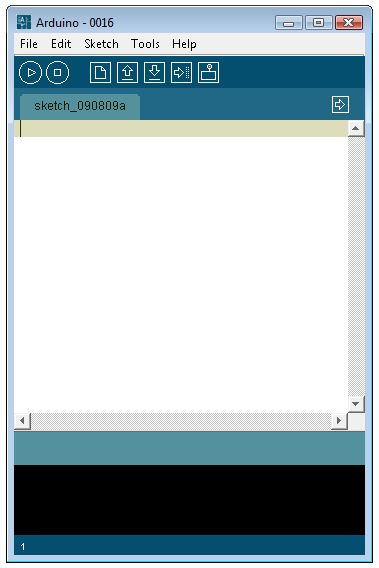• Find the COM Port of the Arduino.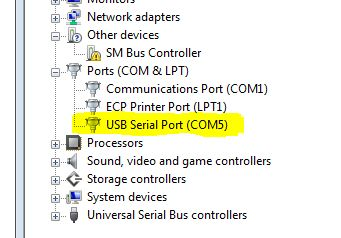• Setting the COM port in Arudino Programe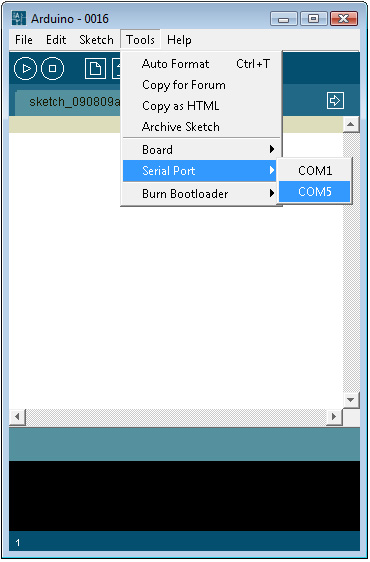### Example 01: Blinking LED . Its the 'Hello World' of Arduino.

• Type out the code.
``````
#define LED 13		// LED connected to
// digitial pin 13.

void setup()
{
pinMode(LED, OUTPUT);
}

void loop()
{
digitalWrite(LED, HIGH);	// turns the LED on
delay(1000);		// waits for a second
digitalWrite(LED, LOW);	// turns the LED off
delay(1000);		// waits for a second
}

``````
• Verify the code.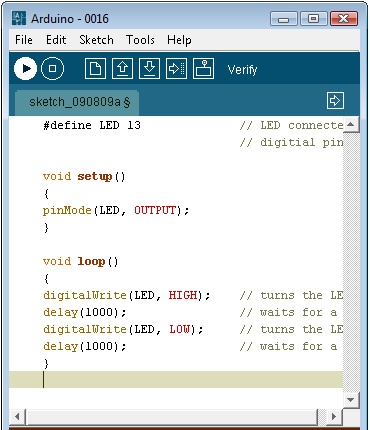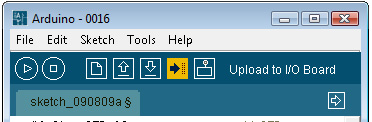• Insert LED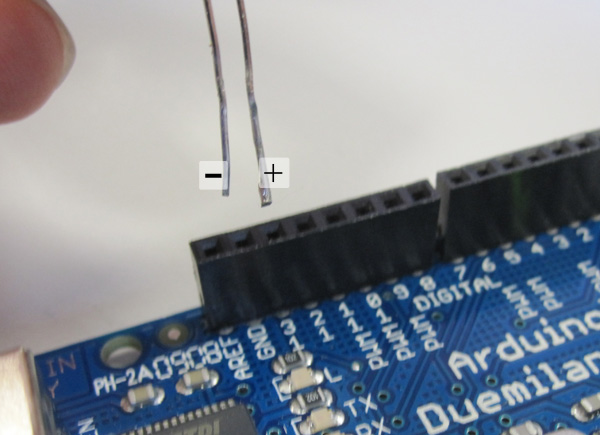### Example 02: Using a Pushbutton to Control the LED.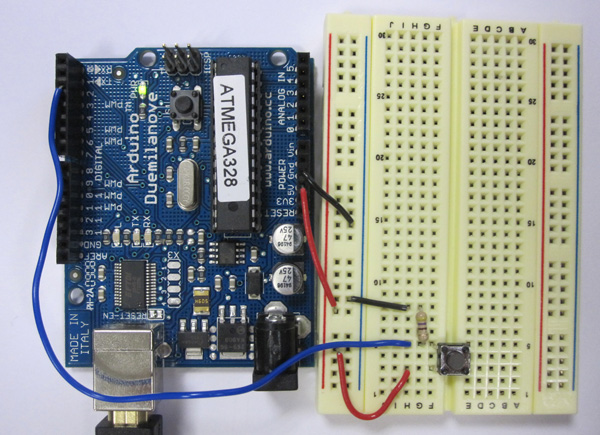``````
#define LED 13		// LED connected to
// digitial pin 13.
#define BUTTON 2 	// in input for the button
int val = 0;	//this value store the button state

void setup() {
pinMode(LED, OUTPUT);
pinMode(BUTTON, INPUT);
}

void loop() {

// Uses an IF statement to see if BUTTON is pressed.
if (val == HIGH ) {
digitalWrite(LED, HIGH); //turn LED On.
} else {
digitalWrite(LED, LOW);
}
}
}
``````

Example 2.

``````
#define LED 13		// LED connected to
// digitial pin 13.
#define BUTTON 2 	// in input for the button
int val = 0;	//this value store the button state
int state = 0;	// 0 = LED off while 1 = LED on.

void setup(){
pinMode(LED, OUTPUT);
pinMode(BUTTON, INPUT);
}

void loop(){

// Uses an IF statement to see if BUTTON is pressed.
// and changes it state
if (val == HIGH {
state = 1 - state;
}

if (state == 1) {
digitalWrite(LED, HIGH);	//Turn LED on.
} else {
digitalWrite(LED, LOW);
}
}
``````

Example 3. Turn on LED when the button is pressed and keep it on after it is released. Including simple de-bouncing.

``````
#define LED 13		// LED connected to
// digitial pin 13.
#define BUTTON 2 	// in input for the button
int val = 0;		//this value store the button state
int state = 0;	// 0 = LED off while 1 = LED on.
int old_val = 0;	// stores the previous val.

void setup(){
pinMode(LED, OUTPUT);
pinMode(BUTTON, INPUT);
}

void loop() {

// Uses an IF statement to see if BUTTON is pressed.
// and changes it state
if ((val == HIGH ) && (old_val == LOw)) {
state = 1 - state;
delay(10);
}

old_val = val; // val is old, and stored in the old_val.

if (state == 1) {
digitalWrite(LED, HIGH);	//Turn LED on.
} else {
digitalWrite(LED, LOW);
}
}
``````

### Example 03:Using PWN to control the LED.An example that fades an LED in and out. Looks like Apple?

``````
#define LED 9		// LED connected to
// digitial pin 9.
int i = 0;		//this value store the button state

void setup(){
pinMode(LED, OUTPUT);
}

void loop(){
for (i = 0; i < 255; i++) { //loops from 0 to 254. Fades Up.
analogWrite(LED, i);
delay(10);	//Wait 10ms because analogWrite would otherwise
// be instantaneous.
}

for (i = 255; i > 0; i--) { //loops from 255 to 1. Fade Down.
analogWrite(LED, i);	// Gives the LED power.
delay(10);
}
}
``````

The more resistance the longer the light will come on.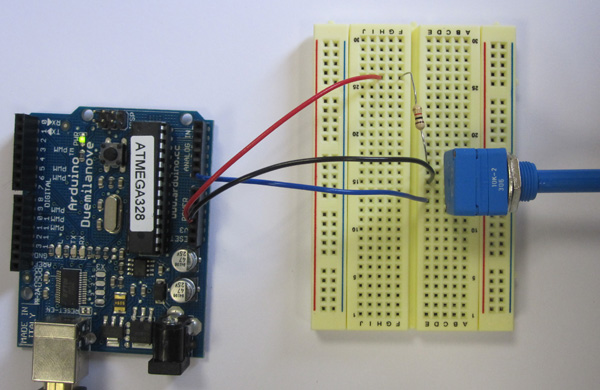`````` /*
turning on and off a light emitting diode(LED)  connected to digital pin 13.
The amount of time the LED will be on and off depends on

The circuit:

*/
int sensorPin = 0;    // select the input pin for the potentiometer
int ledPin = 13;      // select the pin for the LED
int sensorValue = 0;  // variable to store the value coming from the sensor
void setup() {
// declare the ledPin as an OUTPUT:
pinMode(ledPin, OUTPUT);
}
void loop() {
// read the value from the sensor:
// turn the ledPin on
digitalWrite(ledPin, HIGH);
// stop the program for  milliseconds:
delay(sensorValue);
// turn the ledPin off:
digitalWrite(ledPin, LOW);
// stop the program for for  milliseconds:
delay(sensorValue);
}```
```

### Example 05:Sending data to the serial port.

Example 05: Sending data to the serial port.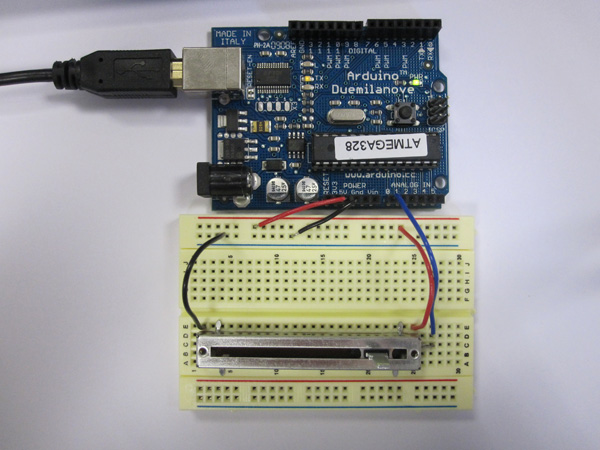``````
#define SENSOR 1
int val = 0;

void setup(){
Serial.begin(9600);   //Opens a serial port.
}

void loop(){